Download Presentation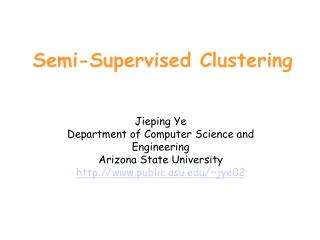Semi-Supervised Clustering

# Semi-Supervised Clustering - PowerPoint PPT Presentation

Semi-Supervised Clustering. Jieping Ye Department of Computer Science and Engineering Arizona State University http://www.public.asu.edu/~jye02. Outline. Overview of clustering and classification What is semi-supervised learning? Semi-supervised clustering Semi-supervised classificationI am the owner, or an agent authorized to act on behalf of the owner, of the copyrighted work described.
Download Presentation## Semi-Supervised Clustering

An Image/Link below is provided (as is) to download presentation

Download Policy: Content on the Website is provided to you AS IS for your information and personal use and may not be sold / licensed / shared on other websites without getting consent from its author.While downloading, if for some reason you are not able to download a presentation, the publisher may have deleted the file from their server.

- - - - - - - - - - - - - - - - - - - - - - - - - - E N D - - - - - - - - - - - - - - - - - - - - - - - - - -
Presentation Transcript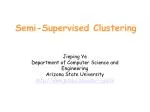### Semi-Supervised Clustering

Jieping Ye

Department of Computer Science and Engineering

Arizona State University

http://www.public.asu.edu/~jye02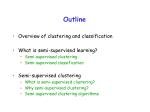Outline
• Overview of clustering and classification
• What is semi-supervised learning?
• Semi-supervised clustering
• Semi-supervised classification
• Semi-supervised clustering
• What is semi-supervised clustering?
• Why semi-supervised clustering?
• Semi-supervised clustering algorithms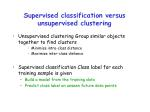Supervised classification versus unsupervised clustering
• Unsupervised clustering Group similar objects together to find clusters
• Minimize intra-class distance
• Maximize inter-class distance
• Supervised classification Class label for each training sample is given
• Build a model from the training data
• Predict class label on unseen future data points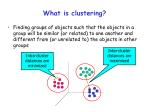Inter-cluster distances are maximized

Intra-cluster distances are minimized

What is clustering?
• Finding groups of objects such that the objects in a group will be similar (or related) to one another and different from (or unrelated to) the objects in other groups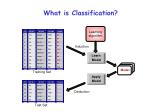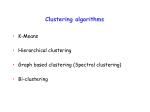Clustering algorithms
• K-Means
• Hierarchical clustering
• Graph based clustering (Spectral clustering)
• Bi-clustering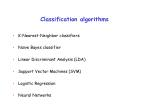Classification algorithms
• K-Nearest-Neighbor classifiers
• Naïve Bayes classifier
• Linear Discriminant Analysis (LDA)
• Support Vector Machines (SVM)
• Logistic Regression
• Neural Networks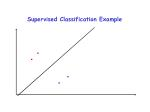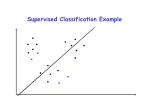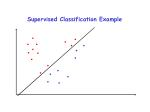Semi-supervised

learning

Supervised

classification

Unsupervised

clustering

Semi-Supervised Learning
• Combines labeled and unlabeled data during training to improve performance:
• Semi-supervised classification: Training on labeled data exploits additional unlabeled data, frequently resulting in a more accurate classifier.
• Semi-supervised clustering: Uses small amount of labeled data to aid and bias the clustering of unlabeled data.Semi-Supervised Classification
• Algorithms:
• Semisupervised EM [Ghahramani:NIPS94,Nigam:ML00].
• Co-training [Blum:COLT98].
• Transductive SVM’s [Vapnik:98,Joachims:ICML99].
• Graph based algorithms
• Assumptions:
• Known, fixed set of categories given in the labeled data.
• Goal is to improve classification of examples into these known categories.Semi-supervised clustering: problem definition
• Input:
• A set of unlabeled objects, each described by a set of attributes (numeric and/or categorical)
• A small amount of domain knowledge
• Output:
• A partitioning of the objects into k clusters (possibly with some discarded as outliers)
• Objective:
• Maximum intra-cluster similarity
• Minimum inter-cluster similarity
• High consistency between the partitioning and the domain knowledgeWhy semi-supervised clustering?
• Why not clustering?
• The clusters produced may not be the ones required.
• Sometimes there are multiple possible groupings.
• Why not classification?
• Sometimes there are insufficient labeled data.
• Potential applications
• Bioinformatics (gene and protein clustering)
• Document hierarchy construction
• News/email categorization
• Image categorizationSemi-Supervised Clustering
• Domain knowledge
• Partial label information is given
• Apply some constraints (must-links and cannot-links)
• Approaches
• Search-based Semi-Supervised Clustering
• Alter the clustering algorithm using the constraints
• Similarity-based Semi-Supervised Clustering
• Alter the similarity measure based on the constraints
• Combination of bothSearch-Based Semi-Supervised Clustering
• Alter the clustering algorithm that searches for a good partitioning by:
• Modifying the objective function to give a reward for obeying labels on the supervised data [Demeriz:ANNIE99].
• Enforcing constraints (must-link, cannot-link) on the labeled data during clustering [Wagstaff:ICML00, Wagstaff:ICML01].
• Use the labeled data to initialize clusters in an iterative refinement algorithm (kMeans,) [Basu:ICML02].Overview of K-Means Clustering
• K-Means is a partitional clustering algorithm based on iterative relocation that partitions a dataset into K clusters.

Algorithm:

Initialize K cluster centers randomly. Repeat until convergence:

• Cluster Assignment Step: Assign each data point x to the cluster Xl, such that L2 distance of x from (center of Xl) is minimum
• Center Re-estimation Step: Re-estimate each cluster center as the mean of the points in that clusterK-Means Objective Function
• Locally minimizes sum of squared distance between the data points and their correspondingcluster centers:
• Initialization of K cluster centers:
• Totally random
• Random perturbation from global mean
• Heuristic to ensure well-separated centersSemi-Supervised K-Means
• Partial label information is given
• Seeded K-Means
• Constrained K-Means
• COP K-MeansSemi-Supervised K-Means for partially labeled data
• Seeded K-Means:
• Labeled data provided by user are used for initialization: initial center for cluster i is the mean of the seed points having label i.
• Seed points are only used for initialization, and not in subsequent steps.
• Constrained K-Means:
• Labeled data provided by user are used to initialize K-Means algorithm.
• Cluster labels of seed data are kept unchanged in the cluster assignment steps, and only the labels of the non-seed data are re-estimated.Seeded K-Means

Use labeled data to find

the initial centroids and

then run K-Means.

The labels for seeded

points may change.Exercise

Compute the clustering using seeded KmeansConstrained K-Means

Use labeled data to find

the initial centroids and

then run K-Means.

The labels for seeded

points will not change.Exercise

Compute the clustering using constrained KmeansCOP K-Means
• COP K-Means [Wagstaff et al.: ICML01] is K-Means with must-link (must be in same cluster) and cannot-link (cannot be in same cluster) constraints on data points.
• Initialization: Cluster centers are chosen randomly, but as each one is chosen any must-link constraints that it participates in are enforced (so that they cannot later be chosen as the center of another cluster).
• Algorithm: During cluster assignment step in COP-K-Means, a point is assigned to its nearest cluster without violating any of its constraints. If no such assignment exists, abort.Illustration

Determine

its label

x

x

Assign to the red classIllustration

Determine

its label

x

x

Assign to the red classIllustration

Determine

its label

x

x

The clustering algorithm failsSimilarity-based semi-supervised clustering
• Alter the similarity measure based on the constraints
• Paper: From Instance-level Constraints to Space-Level Constraints: Making the Most of Prior Knowledge in Data Clustering. D. Klein et al.

Two types of constraints: Must-links and Cannot-links

Clustering algorithm: Hierarchical clusteringOverview of Hierarchical Clustering Algorithm
• Agglomerative versusDivisive
• Basic algorithm of Agglomerative clustering
• Compute the distance matrix
• Let each data point be a cluster
• Repeat
• Merge the two closest clusters
• Update the distance matrix
• Until only a single cluster remains
• Key operation is the update of the distance between two clustersp1

p2

p3

p4

p5

. . .

p1

p2

p3

p4

p5

.

.

.

How to Define Inter-Cluster Distance

Distance?

• MIN
• MAX
• Group Average
• Distance Between Centroids

distance matrix• Distance between must-links pair to zero.
• Derive a new metric by running an all-pairs-shortest distances algorithm.
• It is still a metric
• Faithful to the original metric
• Computational complexity: O(N2C)
• C: number of points involved in must-link constraints
• N: total number of pointsp1

p2

p3

p4

p5

. . .

p1

p2

p3

p4

p5

.

.

.

New distance matrix based on must-link constraints

Hierarchical clustering can be carried out based on the new distance matrix.

What is missing?

New distance matrix• Run hierarchical clustering with complete link (MAX)
• The distance between two clusters is determined by the largest distance.
• Set the distance between cannot-link pair to be
• The new distance matrix does not define a metric.
• Work very well in practiceConstrained complete-link clustering algorithm

Derive a new distance

Matrix based on both

Types of constraintsIllustration

2

1

4

3

5

Initial distance matrixNew distance matrix

0.9Hierarchical clustering

1 and 2 form a cluster, and 3 and 4 form another cluster

1,2

3,4

5

1,2

3,4

1 2 3 4 5

5Summary
• Seeded and Constrained K-Means: partially labeled data
• COP K-Means: constraints (Must-link and Cannot-link)
• Constrained K-Means and COP K-Means require all the constraints to be satisfied.
• May not be effective if the seeds contain noise.
• Seeded K-Means use the seeds only in the first step to determine the initial centroids.
• Less sensitive to the noise in the seeds.
• Semi-supervised hierarchical clustering